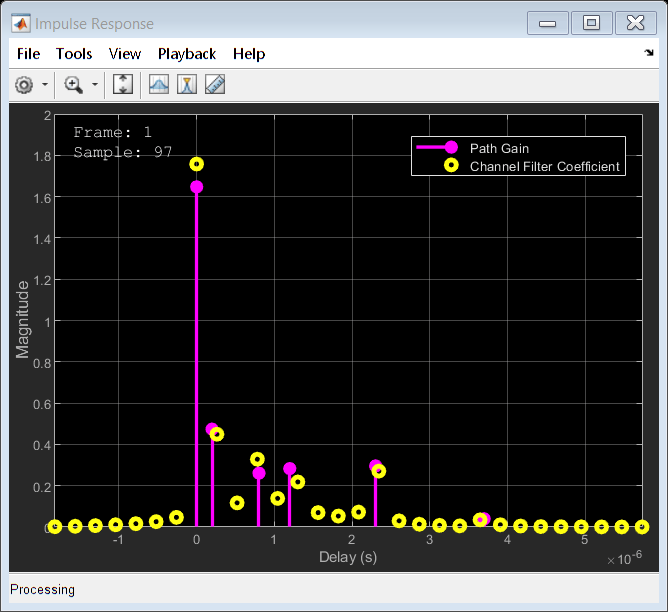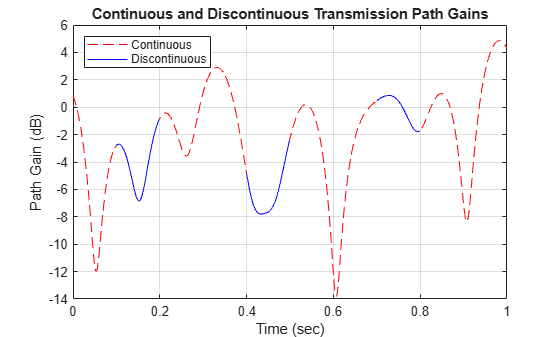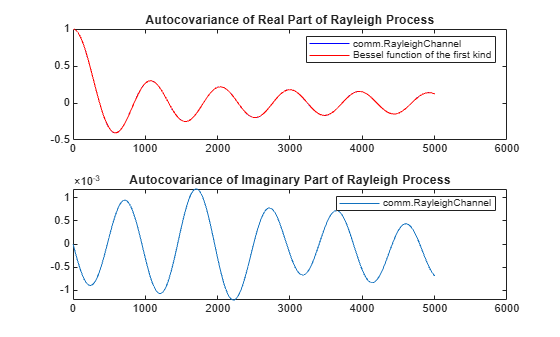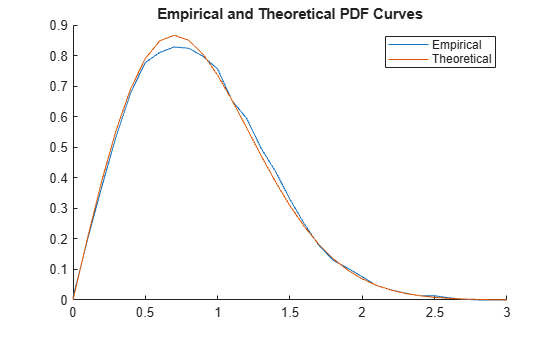# comm.RayleighChannel

Filter input signal through multipath Rayleigh fading channel

## Description

The `comm.RayleighChannel` System object™ filters an input signal through the multipath Rayleigh fading channel. For more information on fading model processing, see the Methodology for Simulating Multipath Fading Channels section.

To filter an input signal through a multipath Rayleigh fading channel:

1. Create the `comm.RayleighChannel` object and set its properties.

2. Call the object with arguments, as if it were a function.

## Creation

### Syntax

``rayleighchan = comm.RayleighChannel``
``rayleighchan = comm.RayleighChannel(Name,Value)``

### Description

````rayleighchan = comm.RayleighChannel` creates a frequency-selective or frequency-flat multipath Rayleigh fading channel System object. This object filters a real or complex input signal through the multipath channel to obtain a channel-impaired signal.```

example

````rayleighchan = comm.RayleighChannel(Name,Value)` sets properties using one or more name-value arguments. For example, `'SampleRate',2` sets the input signal sample rate to 2.```

## Properties

expand all

Unless otherwise indicated, properties are nontunable, which means you cannot change their values after calling the object. Objects lock when you call them, and the `release` function unlocks them.

If a property is tunable, you can change its value at any time.

Input signal sample rate in hertz, specified as a positive scalar.

Data Types: `double`

Discrete path delay in seconds, specified as a scalar or row vector.

• When you set `PathDelays` to a scalar, the channel is frequency flat.

• When you set `PathDelays` to a vector, the channel is frequency selective.

The `PathDelays` and `AveragePathGains` properties must be the same length.

Data Types: `double`

Average gains of the discrete paths in decibels, specified as a scalar or row vector. The `AveragePathGains` and `PathDelays` properties must be the same length.

Data Types: `double`

Normalize average path gains, specified as one of these logical values:

• `1` (`true`) — The fading processes are normalized so that the total power of the path gains, averaged over time, is 0 dB.

• `0` (`false`) — The total power of the path gains is not normalized.

The `AveragePathGains` property specifies the average powers of the path gains.

Data Types: `logical`

Maximum Doppler shift for all channel paths, specified as a nonnegative scalar. Units are in hertz.

The maximum Doppler shift limit applies to each channel path. When you set this property to `0`, the channel remains static for the entire input. You can use the `reset` object function to generate a new channel realization. The `MaximumDopplerShift` property value must be smaller than `SampleRate`/10/fc for each path. fc is the cutoff frequency factor of the path. For most Doppler spectrum types, the value of fc is 1. For Gaussian and bi-Gaussian Doppler spectrum types, fc is dependent on the Doppler spectrum structure fields. For more details about how fc is defined, see the Cutoff Frequency Factor section.

Data Types: `double`

Doppler spectrum shape for all channel paths, specified as a Doppler spectrum structure or a 1-by-NP cell array of Doppler spectrum structures. These Doppler spectrum structures must be outputs of the form returned from the `doppler` function. NP is the number of discrete delay paths specified by the `PathDelays` property. The `MaximumDopplerShift` property defines the maximum Doppler shift value that the `DopplerSpectrum` property permits when you specify the Doppler spectrum..

• When you set `DopplerSpectrum` to a single Doppler spectrum structure, all paths have the same specified Doppler spectrum.

• When you set `DopplerSpectrum` to a cell array of Doppler spectrum structures, each path has the Doppler spectrum specified by the corresponding structure in the cell array.

Specify options for the spectrum type by using the `specType` input to the `doppler` function. If you set the `FadingTechnique` property to `'Sum of sinusoids'`, you must set `DopplerSpectrum` to `doppler('Jakes')`.

#### Dependencies

To enable this property, set the `MaximumDopplerShift` property to a positive scalar.

Data Types: `struct` | `cell`

Channel filtering, specified as one of these logical values:

• `1` (`true`) — The channel accepts an input signal and produces a filtered output signal.

• `0` (`false`) — The object does not accept an input signal, produces no filtered output signal, and outputs only channel path gains. You must specify the duration of the fading process by using the `NumSamples` property.

Data Types: `logical`

Output channel path gains, specified as a logical `0` (`false`) or `1` (`true`). Set this property to `true` to output the channel path gains of the underlying fading process.

#### Dependencies

To enable this property, set the `ChannelFiltering` property to `true`.

Data Types: `logical`

Number of samples used for the duration of the fading process, specified as a nonnegative integer.

Tunable: Yes

#### Dependencies

To enable this property, set the `ChannelFiltering` property to `false`.

Data Types: `double`

Path gain output data type, specified as `'double'` or `'single'`.

#### Dependencies

To enable this property, set the `ChannelFiltering` property to `false`.

Data Types: `char` | `string`

Channel model fading technique, specified as ```'Filtered Gaussian noise'``` or `'Sum of sinusoids'`.

Data Types: `char` | `string`

Number of sinusoids used to model the fading process, specified as a positive integer.

#### Dependencies

To enable this property, set the `FadingTechnique` property to `'Sum of sinusoids'`.

Data Types: `double`

Source to control the start time of the fading process, specified as `'Property'` or `'Input port'`.

• When you set `InitialTimeSource` to `'Property'`, set the initial time offset by using the `InitialTime` property.

• When you set `InitialTimeSource` to ```'Input port'```, specify the start time of the fading process by using the `inittime` input argument. The input value can change in consecutive calls to the object.

#### Dependencies

To enable this property, set the `FadingTechnique` property to `'Sum of sinusoids'`.

Data Types: `char` | `string`

Initial time offset for the fading model in seconds, specified as a nonnegative scalar.

When `mod`(`InitialTime`/`SampleRate`) is nonzero, the initial time offset is rounded up to the nearest sample position.

#### Dependencies

To enable this property, set the `FadingTechnique` property to `'Sum of sinusoids'` and the `InitialTimeSource` property to `'Property'`.

Data Types: `double`

Source of the random number stream, specified as ```'Global stream'``` or `'mt19937ar with seed'`.

• When you specify `'Global stream'`, the object uses the current global random number stream for random number generation. In this case, the `reset` object function resets only the filters.

• When you specify `'mt19937ar with seed'`, the object uses the mt19937ar algorithm for random number generation. In this case, the `reset` object function resets the filters and reinitializes the random number stream to the value of the `Seed` property.

Data Types: `char` | `string`

Initial seed of the mt19937ar random number stream generator algorithm, specified as a nonnegative integer. When you call the `reset` object function, it reinitializes the mt19937ar random number stream to the `Seed` value.

#### Dependencies

To enable this property, set the `RandomStream` property to `'mt19937ar with seed'`.

Data Types: `double`

Channel visualization, specified as `'Off'`, ```'Impulse response'```, `'Frequency response'`, ```'Impulse and frequency responses'```, or `'Doppler spectrum'`. For more information, see the Channel Visualization topic.

#### Dependencies

To enable this property, set the `FadingTechnique` property to `'Filtered Gaussian noise'`.

Data Types: `char` | `string`

Path used for displaying the Doppler spectrum, specified as a positive integer in the range [1, NP]. NP is the number of discrete delay paths specified by the `PathDelays` property. Use this property to select the discrete path used in constructing a Doppler spectrum plot.

#### Dependencies

To enable this property, set the `Visualization` property to `'Doppler spectrum'`.

Data Types: `double`

Percentage of samples to display, specified as `'25%'`, `'10%'`, `'50%'`, or `'100%'`. Increasing the percentage improves display accuracy at the expense of simulation speed.

#### Dependencies

To enable this property, set the `Visualization` property to `'Impulse response'`, ```'Frequency response'```, or ```'Impulse and frequency responses'```.

Data Types: `char` | `string`

## Usage

### Syntax

``y = rayleighchan(x)``
``y = rayleighchan(x,inittime)``
``[y,pathgains] = rayleighchan(___)``
``pathgains = rayleighchan()``
``pathgains = rayleighchan(inittime)``

### Description

example

````y = rayleighchan(x)` filters the input signal `x` through a multipath Rayleigh fading channel and returns the result in `y`. To enable this syntax, set the `ChannelFiltering` property to `true`.```

example

````y = rayleighchan(x,inittime)` specifies a start time for the fading process.To enable this syntax, set the `FadingTechnique` property to `'Sum of sinusoids'` and the `InitialTimeSource` property to `'Input port'`.```

example

````[y,pathgains] = rayleighchan(___)` also returns the channel path gains of the underlying multipath Rayleigh fading process in `pathgains` using any of the input argument combinations in the previous syntaxes.To enable this syntax, set the `PathGainsOutputPort` property set to `true`.```

example

````pathgains = rayleighchan()` returns the channel path gains of the underlying fading process. In this case, the channel requires no input signal and acts as a source of path gains.To enable this syntax, set the `ChannelFiltering` property to `false`.```
````pathgains = rayleighchan(inittime)` returns the channel path gains of the underlying fading process beginning at the specified initial time. In this case, the channel requires no input signal and acts as a source of path gains.To enable this syntax, set the `FadingTechnique` property to `'Sum of sinusoids'`, the `InitialTimeSource` property to `'Input port'`, and the `ChannelFiltering` property to `false`.```

### Input Arguments

expand all

Input signal, specified as an NS-by-1 vector, where NS is the number of samples.

Data Types: `single` | `double`
Complex Number Support: Yes

Initial time offset in seconds, specified as a nonnegative scalar.

When `mod`(`inittime`/`SampleRate`) is nonzero, the initial time offset is rounded up to the nearest sample position.

Data Types: `single` | `double`

### Output Arguments

expand all

Output signal, returned as an NS-by-1 vector of complex values with the same data precision as the input signal `x`. NS is the number of samples.

Output path gains, returned as an NS-by-NP matrix. NS is the number of samples. NP is the number of discrete delay paths specified by the `PathDelays` property. `pathgains` contains complex values.

When you set the `ChannelFiltering` property to `false`, the data type of this output has the same precision as the input signal `x`. When you set the `ChannelFiltering` property to `true`, the data type of this output is specified by the `OutputDataType` property.

## Object Functions

To use an object function, specify the System object as the first input argument. For example, to release system resources of a System object named `obj`, use this syntax:

`release(obj)`

expand all

 `info` Characteristic information about fading channel object
 `step` Run System object algorithm `release` Release resources and allow changes to System object property values and input characteristics `reset` Reset internal states of System object

## Examples

collapse all

Produce the same multipath Rayleigh fading channel response by using two different methods for random number generation. The multipath Rayleigh fading channel System object includes two methods for random number generation. You can use the current global stream or the mt19937ar algorithm with a specified seed. By interacting with the global stream, the System object can produce the same outputs from these two methods.

Create a PSK modulator System object to modulate randomly generated data.

```pskModulator = comm.PSKModulator; insig = randi([0,pskModulator.ModulationOrder-1],1024,1); channelInput = pskModulator(insig);```

Create a multipath Rayleigh fading channel System object, specifying the random number generation method as the my19937ar algorithm and the random number seed as 22.

```rayleighchan = comm.RayleighChannel( ... 'SampleRate',10e3, ... 'PathDelays',[0 1.5e-4], ... 'AveragePathGains',[2 3], ... 'NormalizePathGains',true, ... 'MaximumDopplerShift',30, ... 'DopplerSpectrum',{doppler('Gaussian',0.6),doppler('Flat')}, ... 'RandomStream','mt19937ar with seed', ... 'Seed',22, ... 'PathGainsOutputPort',true);```

Filter the modulated data by using the multipath Rayleigh fading channel System object.

`[chanOut1,pathGains1] = rayleighchan(channelInput);`

Set the System object to use the global stream for random number generation.

```release(rayleighchan); rayleighchan.RandomStream = 'Global stream';```

Set the global stream to have the same seed that you specified when creating the multipath Rayleigh fading channel System object.

`rng(22)`

Filter the modulated data by using the multipath Rayleigh fading channel System object again.

`[chanOut2,pathGains2] = rayleighchan(channelInput);`

Verify that the channel and path gain outputs are the same for each of the two methods.

`isequal(chanOut1,chanOut2)`
```ans = logical 1 ```
`isequal(pathGains1,pathGains2)`
```ans = logical 1 ```

Display the impulse and frequency responses of a frequency-selective multipath Rayleigh fading channel that is configured to disable channel filtering.

Define simulation variables. Specify path delays and gains by using the ITU pedestrian B channel configuration.

```fs = 3.84e6; % Sample rate in Hz pathDelays = [0 200 800 1200 2300 3700]*1e-9; % in seconds avgPathGains = [0 -0.9 -4.9 -8 -7.8 -23.9]; % dB fD = 50; % Max Doppler shift in Hz```

Create a multipath Rayleigh fading channel System object to visualize the impulse response and frequency response plots.

```rayleighchan = comm.RayleighChannel('SampleRate',fs, ... 'PathDelays',pathDelays, ... 'AveragePathGains',avgPathGains, ... 'MaximumDopplerShift',fD, ... 'ChannelFiltering',false, ... 'Visualization','Impulse and frequency responses');```

Visualize the channel response by running the multipath Rayleigh fading channel System object with no input signal. The impulse response plot enables you to identify the individual paths and their corresponding filter coefficients. The frequency response plot shows the frequency-selective nature of the ITU pedestrian B channel.

`rayleighchan();`Show that the channel state is maintained for discontinuous transmissions by using multipath Rayleigh fading channel System objects that use the sum-of-sinusoids technique. Observe discontinuous channel response segments overlaid on a continuous channel response.

Set the channel properties.

```fs = 1000; % Sample rate (Hz) pathDelays = [0 2.5e-3]; % In seconds pathPower = [0 -6]; % In dB fD = 5; % Maximum Doppler shift (Hz) ns = 1000; % Number of samples nsdel = 100; % Number of samples for delayed paths```

Define a continuous time span and three discontinuous time segments over which to plot and view the channel response. View a 1000-sample continuous channel response that starts at time 0 and three 100-sample channel responses that start at times 0.1, 0.4, and 0.7 seconds, respectively.

```to0 = 0.0; to1 = 0.1; to2 = 0.4; to3 = 0.7; t0 = (to0:ns-1)/fs; % Transmission 0 t1 = to1+(0:nsdel-1)/fs; % Transmission 1 t2 = to2+(0:nsdel-1)/fs; % Transmission 2 t3 = to3+(0:nsdel-1)/fs; % Transmission 3```

Create a frequency-flat multipath Rayleigh fading System object, specifying a 1000 Hz sampling rate, the sum-of-sinusoids fading technique, disabled channel filtering, and the number of samples to view. Specify a seed value so that results can be repeated. Use the default `InitialTime` property setting so that the fading channel is simulated from time 0.

```rayleighchan1 = comm.RayleighChannel('SampleRate',fs, ... 'MaximumDopplerShift',fD, ... 'RandomStream','mt19937ar with seed', ... 'Seed',17, ... 'FadingTechnique','Sum of sinusoids', ... 'ChannelFiltering',false, ... 'NumSamples',ns);```

Create a clone of the multipath Rayleigh fading channel System object. In the cloned object, set the number of samples to view for the delayed paths. Also configure the initial time source as an input so that you can specify the fading channel offset time as an input argument when using the System object.

```rayleighchan2 = clone(rayleighchan1); rayleighchan2.NumSamples = nsdel; rayleighchan2.InitialTimeSource = 'Input port';```

Save the path gain output for the continuous channel response by using the `rayleighchan1` object and for the discontinuous delayed channel responses by using the `rayleighchan2` object with initial time offsets are provided as input arguments.

```pg0 = rayleighchan1(); pg1 = rayleighchan2(to1); pg2 = rayleighchan2(to2); pg3 = rayleighchan2(to3);```

Compare the number of samples processed by the two channels by using the `info` object function. The `rayleighchan1` object processed 1000 samples, while the `rayleighchan2` object processed only 300 samples.

```G = info(rayleighchan1); H = info(rayleighchan2); [G.NumSamplesProcessed H.NumSamplesProcessed]```
```ans = 1×2 1000 300 ```

Convert the path gains into decibels.

```pathGain0 = 20*log10(abs(pg0)); pathGain1 = 20*log10(abs(pg1)); pathGain2 = 20*log10(abs(pg2)); pathGain3 = 20*log10(abs(pg3));```

Plot the path gains for the continuous and discontinuous cases. The gains for the three segments match the gain for the continuous case. Because the channel characteristics are maintained even when data is not transmitted, the alignment of the two plots shows that the sum-of-sinusoids technique is suited to the simulation of packetized data.

```plot(t0,pathGain0,'r--') hold on plot(t1,pathGain1,'b') plot(t2,pathGain2,'b') plot(t3,pathGain3,'b') grid xlabel('Time (sec)') ylabel('Path Gain (dB)') legend('Continuous','Discontinuous','location','nw') title('Continuous and Discontinuous Transmission Path Gains')```Reproduce the multipath Rayleigh fading channel output across multiple frames by using the `ChannelFilterCoefficients` property returned by the info object function of the `comm.RayleighChannel` System object.

Create a multipath Rayleigh fading channel System object, defining two paths. Generate data to pass through the channel.

```rayleighchan = comm.RayleighChannel( ... 'SampleRate',1000, ... 'PathDelays',[0 1.5e-3], ... 'AveragePathGains',[0 -3], ... 'PathGainsOutputPort',true)```
```rayleighchan = comm.RayleighChannel with properties: SampleRate: 1000 PathDelays: [0 0.0015] AveragePathGains: [0 -3] NormalizePathGains: true MaximumDopplerShift: 1.0000e-03 DopplerSpectrum: [1x1 struct] ChannelFiltering: true PathGainsOutputPort: true Show all properties ```
`data = randi([0 1],600,1);`

Pass data through the channel. Assign the `ChannelFilterCoefficients` property value to the variable `coeff`. Within a `for` loop, calculate the fractional delayed input signal at the path delay locations stored in `coeff`, apply the path gains, and sum the results for all of the paths. Compare the output of the multipath Rayleigh fading channel System object (`chanout1`) to the output reproduced using the path gains and the `ChannelFilterCoefficients` property of the multipath Rayleigh fading channel System object (`chanout2`).

```chaninfo = info(rayleighchan); coeff = chaninfo.ChannelFilterCoefficients; Np = length(rayleighchan.PathDelays); state = zeros(size(coeff,2)-1,size(coeff,1)); nFrames = 10; chkChan = zeros(nFrames,1); for jj = 1 : nFrames data = randi([0 1],600,1); [chanout1,pg] = rayleighchan(data); fracdelaydata = zeros(size(data,1),Np); % Calculate the fractional delayed input signal. for ii = 1:Np [fracdelaydata(:,ii),state(:,ii)] = ... filter(coeff(ii,:),1,data,state(:,ii)); end % Apply the path gains and sum the results for all of the paths. % Compare the channel outputs. chanout2 = sum(pg .* fracdelaydata,2); chkChan(jj) = isequal(chanout1,chanout2); end chkChan'```
```ans = 1×10 1 1 1 1 1 1 1 1 1 1 ```

Verify that the autocorrelation of the path gain output from the Rayleigh channel System object is a Bessel function. The results in [ 1 ] and Appendix A of [ 2 ], show that when the autocorrelation of the path gain outputs is a Bessel function, the Doppler spectrum is Jakes-shaped.

Initialize simulation parameters.

```Rsym = 9600; % Input symbol rate (symbols/s) sps = 10; % Number of samples per input symbol Fs = sps*Rsym; % Input sampling frequency (samples/s) Ts = 1/Fs; % Input sampling period (s) numsym = 1e6; % Number of input symbols to simulate numsamp = numsym*sps; % Number of channel samples to simulate fd = 100; % Maximum Doppler frequency shift (Hz) num_acsamp = 5000; % Number of samples of autocovariance % of complex fading process calculated numtx = 1; % Number of transmit antennas numrx = 1; % Number of receive antennas numsin = 48; % Number of sinusoids frmLen = 10000; numFrames = numsamp/frmLen;```

Configure a Rayleigh channel System object.

```chan = comm.RayleighChannel( ... 'FadingTechnique','Sum of sinusoids', ... 'NumSinusoids',numsin, ... 'RandomStream','mt19937ar with seed', ... 'PathDelays',0, ... 'AveragePathGains',0, ... 'SampleRate',Fs, ... 'MaximumDopplerShift',fd, ... 'PathGainsOutputPort',true);```

Apply DPSK modulation to a random bit stream.

```tx = randi([0 1],numsamp,numtx); % Random bit stream dpskSig = dpskmod(tx,2); % DPSK signal ```

Pass the modulated signal through the channel.

```outsig = zeros(numsamp,numrx); pg_rx = zeros(numsamp,numrx,numtx); for frmNum = 1:numFrames [outsig((1:frmLen)+(frmNum-1)*frmLen,:),pathGains] = ... chan(dpskSig((1:frmLen)+(frmNum-1)*frmLen,:)); for i = 1:numrx pg_rx((1:frmLen)+(frmNum-1)*frmLen,i,:) = ... pathGains(:,:,:,i); end end ```

Using the channel path gains received per antenna, compute the autocovariance of the fading process for each transmit-receive path.

```autocov = zeros(frmLen+1,numrx,numtx); autocov_normalized_real = zeros(num_acsamp+1,numrx,numtx); autocov_normalized_imag = zeros(num_acsamp+1,numrx,numtx); for i = 1:numrx % Compute autocovariance of simulated complex fading process for j = 1:numtx autocov(:,i,j) = xcov(pg_rx(:,i,j),num_acsamp); % Real part of normalized autocovariance autocov_normalized_real(:,i,j) = ... real(autocov(num_acsamp+1:end,i,j) ... / autocov(num_acsamp+1,i,j)); % Imaginary part of normalized autocovariance autocov_normalized_imag(:,i,j) = ... imag(autocov(num_acsamp+1:end,i,j) ... / autocov(num_acsamp+1,i,j)); end end ```

Compute the theoretical autocovariance of the complex fading process by using the `besselj` function.

```Rrr = zeros(1,num_acsamp+1); for n = 1:1:num_acsamp+1 Rrr(n) = besselj(0,2*pi*fd*(n-1)*Ts); end Rrr_normalized = Rrr/Rrr(1); ```

Display the autocovariance to compare the results from the Rayleigh channel System object and the `besselj` function.

```subplot(2,1,1) plot(autocov_normalized_real,'b-') hold on plot(Rrr_normalized,'r-') hold off legend('comm.RayleighChannel', ... 'Bessel function of the first kind') title('Autocovariance of Real Part of Rayleigh Process') subplot(2,1,2) plot(autocov_normalized_imag) legend('comm.RayleighChannel') title('Autocovariance of Imaginary Part of Rayleigh Process') ```As computed below, the mean square error comparing the results from the Rayleigh channel object versus the Bessel function is insignificant.

```act_mse_real = ... sum((autocov_normalized_real-repmat(Rrr_normalized.',1,numrx,numtx)).^2,1) ... / size(autocov_normalized_real,1) ```
```act_mse_real = 7.0043e-08 ```
```act_mse_imag = sum((autocov_normalized_imag-0).^2,1) ... / size(autocov_normalized_imag,1)```
```act_mse_imag = 4.1064e-07 ```

References

1. Dent, P., G.E. Bottomley, and T. Croft. “Jakes Fading Model Revisited.” Electronics Letters 29, no. 13 (1993): 1162. https://doi.org/10.1049/el:19930777.

2. Pätzold, Matthias. Mobile Fading Channels. Chichester, UK: John Wiley & Sons, Ltd, 2002. https://doi.org/10.1002/0470847808.

Compute and plot the empirical and theoretical probability density function (PDF) for a Rayleigh channel with one path.

Initialize parameters and create a Rayleigh channel System object that does not apply channel filtering.

```Ns = 1.92e6; Rs = 1.92e6; dopplerShift = 2000; chan = comm.RayleighChannel( ... 'SampleRate',Rs, ... 'PathDelays',0, ... 'AveragePathGains',0, ... 'MaximumDopplerShift',dopplerShift, ... 'ChannelFiltering',false, ... 'NumSamples',Ns, ... 'FadingTechnique','Sum of sinusoids');```

Compute and plot the empirical and theoretical PDF for the Rayleigh channel, by using the `fitdist` (Statistics and Machine Learning Toolbox) and `pdf` (Statistics and Machine Learning Toolbox) functions.

```figure; hold on; % Empirical PDF plot gain = chan(); pd = fitdist(abs(gain),'Kernel','BandWidth',.01); r = 0:.1:3; y = pdf(pd,r); plot(r,y) % Theoretical PDF plot exp_pdf_amplitude = raylpdf(r,0.7); plot(r,exp_pdf_amplitude') legend('Empirical','Theoretical') title('Empirical and Theoretical PDF Curves')```